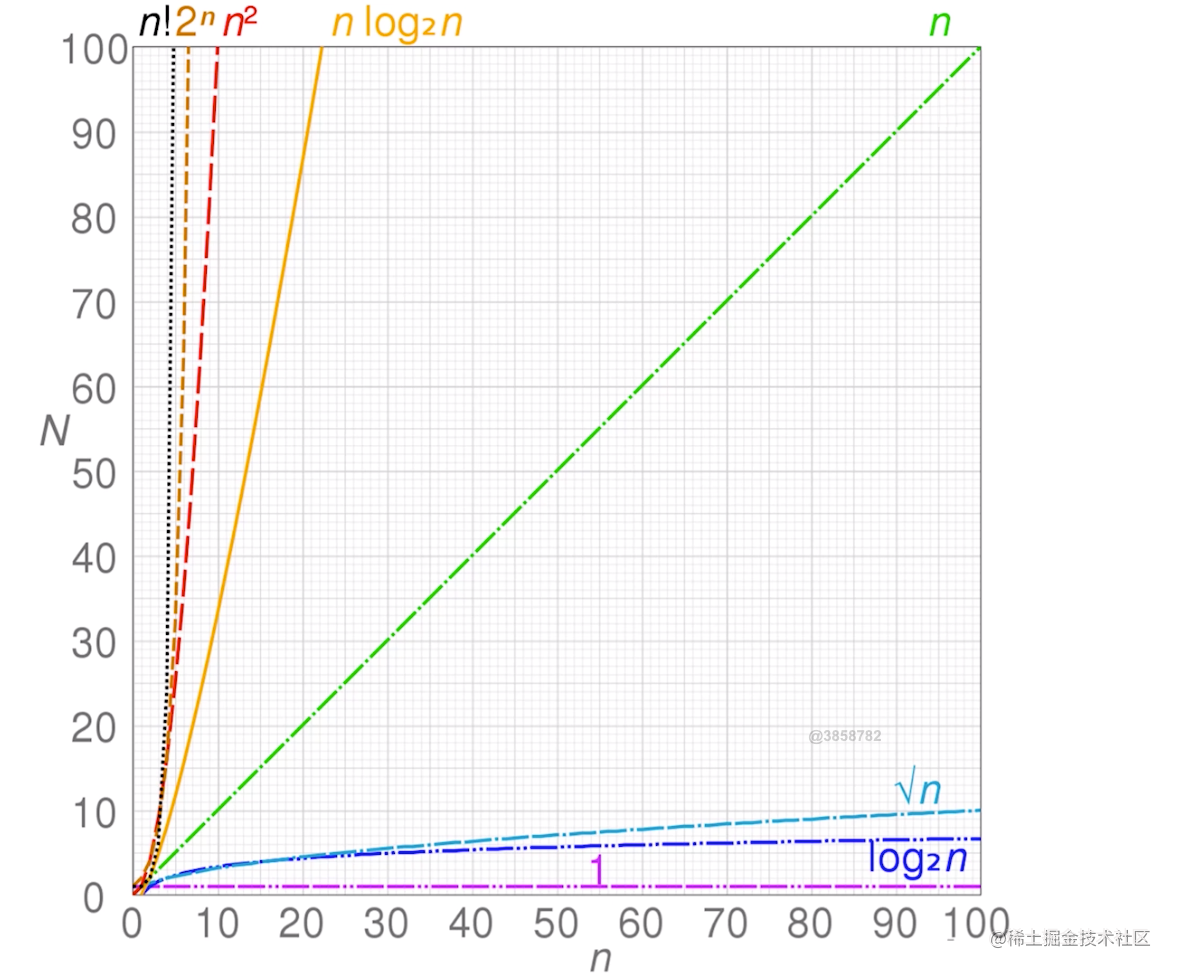算法入门：时间复杂度和空间复杂度及其计算1.大o表示法:

• 算法的运行时间不是以秒为单位的。
• 算法的运行时间是从增速的角度度量的。
• 算法的运行时间用大O表示法表示。

2.时间复杂度：2.1 时间复杂度O(1):

let a = 996;
a +=1;

• 每次执行代码的时候，上面的两行代码只会被执行一次，所以时间复杂度为O(1)

2.2 时间复杂度为O(n):

for(let i=0;i < n; i++){
console.log(n)
}

• 上面代码是一个简单的循环，for循环中的代码被执行了n次时间复杂度为O(n);

2.3 时间复杂度O(n^2):

for(let i=0;i < n; i++){
for(let j=0;j < n; j++){
console.log(i,j);
}
}

• 两层for循环，也就是循环中套循环的

2.4 时间复杂度为O(logN):

logN是啥意思呢？

let i = 1;
while(i < N){
console.log(i);
i *= 2;
}

• 上述代码是while循环，就是一个数不断地乘以2,直到这个结果大于N,循环停止，代码执行了logN

3.空间复杂度:

3.2空间复杂度 O(n):

let arr = [];
for(let i = 0; i < 0; i+=1){
arr.push(i);
}

• 占用了n个内存单元，所以这段代码的空间复杂度为O(n);

3.3空间复杂度 O(n^2):

let square=[];
for(let i=0;i < n; i++){
square.push([]);
for(let j=0;j < n; j++){
square[i].push(j)
}
}

• 二位数组，就是一个矩阵(行列)